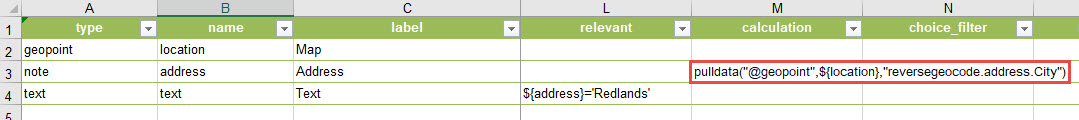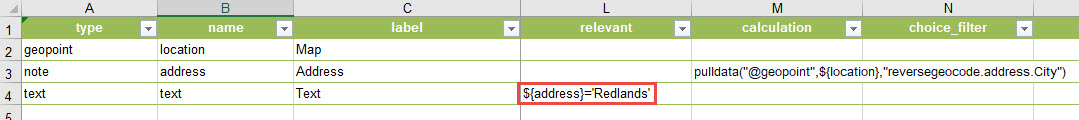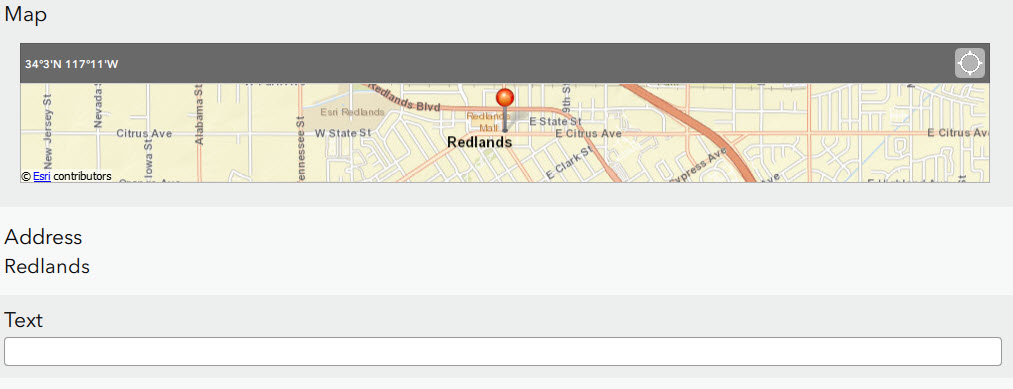English

How To: Display questions based on the geopoint question in Survey123 Connect for ArcGIS

Summary

When creating a survey in Survey123 Connect for ArcGIS, it is possible to control the visibility of a question in the survey based on the value of a geopoint question. For example, when a question must be shown if the geopoint is in a specific city.

Procedure

To tie the visibility of a question based on the value of a geopoint question, use the pulldata and reverse geocoding functions on a note question type to find the location of the geopoint question. Then set the desired location in the relevant column of the question tied to the location. The pulldata reverse geocode can be based on either an address or other parameters created in a reverse geocode, such as the city.

For example, with a geopoint in Redlands, create a note question type in the survey that pulls the city from the reverse geocoded address of the geopoint. Use the following calculation in the calculation column for the note question type.This pulls the city name of the reverse geocoded address. In the question that becomes visible based on the geopoint location, add this statement to the relevant column.By this configuration of the survey, the text question type would only be visible if the value of the note question type is Redlands.The value used from the geocode can be other parameters, such as using the matched address reverse geocode output with the following calculation in the calculation column of the note question type: